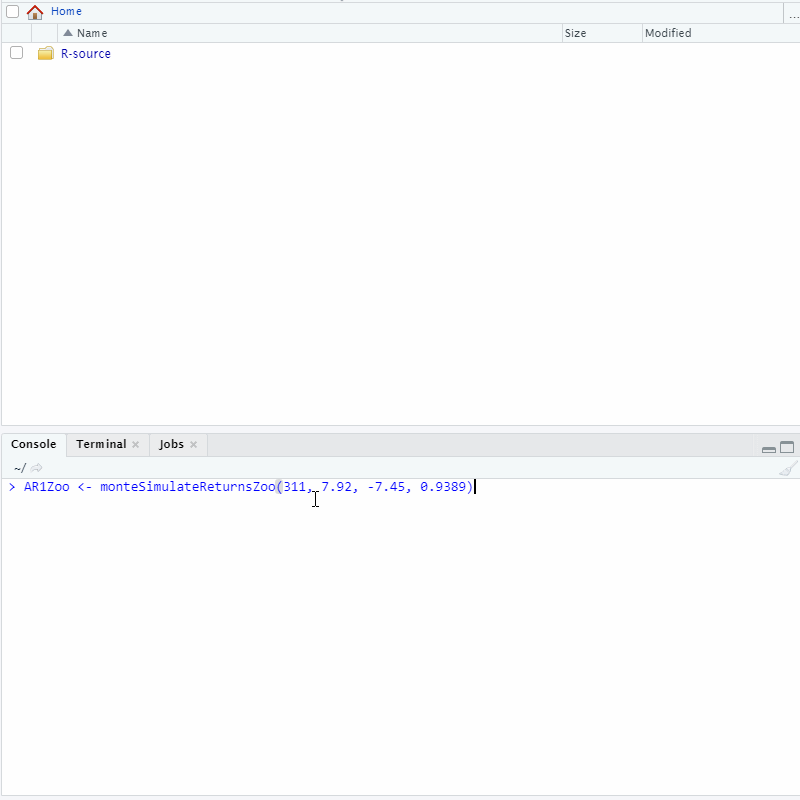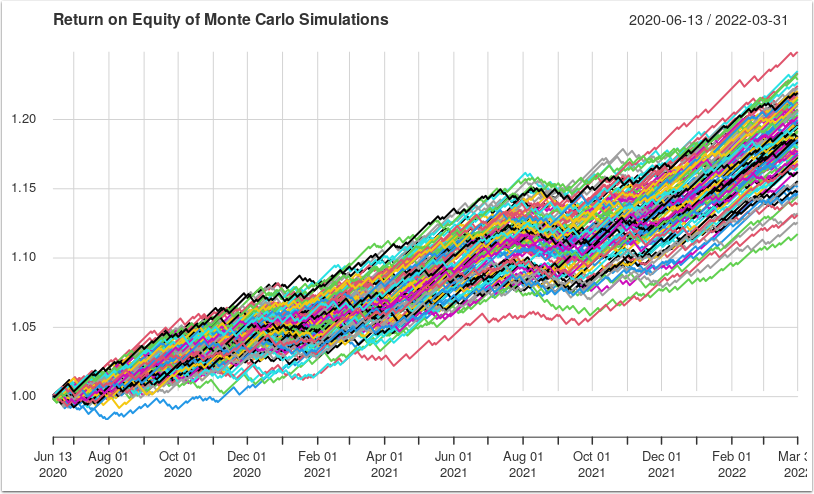## Introduction

TL;DR

We perform a comparative analysis of two Metatrader Signal Providers. The initial Monte Carlo Analysis shows that the first signal provider has a significantly higher return. However, we will show you that an extended MCA, which account for slippages, changes the ranking of the signal providers and ensures a realistic simulation and analysis of returns.

Stylized Facts

• When evaluating a signal provider, a higher average profit ensures a sufficient buffer for slippages.

• An extended Monte Carlo Analysis, which account for slippages, ensures a realistic simulation and analysis of returns.

• As we will show below, an extended MCA may change the ranking of the signal providers, which in turn affects your portfolio.

## Step 1 - Get Statistics

In order to perform a Monte Carlo Analysis ["MCA"] of a Signal Provider, we require several inputs:

• Avg Profit (\$)

• Avg Loss (\$)

• Win Percentage

Let us do a comparative analysis of Automated Robot 1 ["AR1"] and Automated Trading Robot 2 ["ATR2"].

Statistics of AR1

• Avg Profit (\$): 7.92

• Avg Loss (\$): -7.48

• Win Percentage (decimal): 0.9389

Statistics of ATR2

• Avg Profit (\$): 10.79

• Avg Loss (\$): -8.29

• Win Percentage (decimal): 0.9452

The MCA function requires a time series of returns (zoo object) as input, hence we need to simulate the returns, given the statistics above.

The function monteSimulateReturnsZoo() returns a time series of returns (zoo object) and accepts FIVE inputs:

• pAvgNum - a double for average profit in dollars

• lAvgNum - a double for average loss in dollars

• wPctNum - a double for winning percentage in decimal

• initNum - a double for initial capital in dollars (default: 10000)

Type the following command in R to simulate returns for both AR1 and ATR2:

`````` > AR1Zoo <- monteSimulateReturnsZoo( 311, 7.92, -7.45, 0.9389 )
> ATR2Zoo <- monteSimulateReturnsZoo( 657, 10.79, -8.29, 0.9452 )``````

## Step 2 - Monte Carlo Analysis

A Monte Carlo Analysis is a series of random walk forward returns that shows a set of possible outcomes. Each line represents a possible return of a client who follows the given signal provider. Hence, if there are a hundred lines, then these represents 100 possible returns of each client.

We use MCA to look at the worst and average lines from all possible returns to evaluate a signal provider.

For example, from the worst case line, we can estimate the worst case drawdown of our portfolio. If this percentage is too high for our risk appetite, then we should not follow the signal.

Next, we look at the average line, where we can expect to get a similar return for our portfolio. Hence, we should estimate our initial capital to ensure a significant average profit, net of fees. In other words, if the average net profit isn't sufficient, then we should not follow the signal or we could increase our initial capital.

As the MCA is a series of random walk forward returns, each set may not cover all possible outcomes in the universe. Hence, when we look at the worst or average lines from a set, we are only looking within a subset of the whole universe.

The output of the MCA is only as good as the inputs. We perform an Extended MCA to allow for slippages, which affect the outcomes.

MCA in R

The function MonteGrowReturns() returns a Monte object and accepts SEVEN inputs:

• rawZoo - a zoo object of time series returns

• setNum - an integer of number of lines

• sizeNum - an integer of block size, where blocks are kept together (default: 1)

• initNum - a double for initial capital in dollars (default: 10000)

• replaceBln - a boolean for sampling replacement (default: TRUE)

• summary - a boolean for summary (default: TRUE)

• debug - a boolean for debugging (default: FALSE)

Type the following command in R to perform MCA for both AR1 and ATR2:

`````` > AR1Monte <- MonteGrowReturns(AR1Zoo, 100)
> ATR2Monte <- MonteGrowReturns(ATR2Zoo, 100)
> plot(AR1Monte)
> plot(ATR2Monte)``````

Note: At writing, there appears to be an error in the summary, hence we set summary to FALSE. Otherwise, we should accept all other default values.

Results

`````` # Summary of AR1
Initial equity balance    : 10000.00
Absolute maximum drawdown :   -51.33 ( -0.4%) [set = 99]
Absolute median drawdown  :   -25.87 ( -0.2%)
Absolute minimum drawdown :   -14.90 ( -0.1%) [set = 88]
Maximum equity balance    : 12341.67 ( 23.4%) [set = 70]
Median equity balance     : 12157.23 ( 21.6%)
Minimum equity balance    : 11960.57 ( 19.6%) [set = 99]``````

The MCA plot of AR1 (shown below) appears to have higher returns (between 19.6% and 23.4% over 10 months) for all its possible outcomes, compared to the returns for the MCA plot of ATR2.## Step 3 - Get Extended Statistics

In order to perform a more accurate MCA, we need to account for slippages, hence we require further inputs:

• Worst Broker Slippage (pip)

• Total Profit (\$) = Gross Profit (\$) + Gross Loss (\$)

• Total Profit (pip) = Gross Profit (pip) + Gross Loss (pip)

• Pip to Dollar (decimal)

We calculate the Pip to Dollar conversion factor as follows:

``   Pip to Dollar = Total Profit (pip) / Total Profit (\$)``
• Worst Broker Slippage (\$)

We calculate the Worst Broker Slippage (\$) as follows:

``````   Worst Broker Slippage (\$) = Pip to Dollar * Worst Broker Slippage (pip)
Adjusted Avg Profit (\$)   = Avg Profit (\$) - Worst Broker Slippage (\$)
Adjusted Avg Loss (\$)     = Avg Loss (\$) - Worst Broker Slippage (\$)``````
• Percent Decrease (decimal)

After adjusting both the average profit and loss for slippage, it follows that the winning percentage should decrease. However, we don't have the exact number of profitable trades that are less than slippage, e.g. profit of 1.54 will be a loss after slippage of 1.74.

We estimate the Percentage Decrease (decimal) as follows:

``````   Percentage Decrease (decimal)     = Worst Broker Slippage (\$) / Avg Profit (\$)
Adjusted Win Percentage (decimal) = Win Percentage * (1 - Percentage Decrease)``````

Extended Statistics of AR1

• Worst Broker Slippage (pip): 21.7

• Total Profit (\$) = 2313.92 - 141.48 = \$2,172.44

• Total Profit (pip) = 27607 - 548 = 27,049

• Pip to Dollar (decimail) = 2172.44 / 27049 = 0.080315

• Worst Broker Slippage (\$) = 0.080315 * 21.7 = \$1.74

• Percentage Decrease (decimal) = 1.74 / 7.92 = 0.22

• Adj Avg Profit (\$): 7.92 - 1.74 = 6.18

• Adj Avg Loss (\$): -7.48 - 1.74 = -9.22

• Adj Win Percentage (decimal) = (1 - 0.22) * 0.9389 = 0.7323

Extended Statistics of ATR2

• Worst Broker Slippage (pip): 21.7

• Total Profit (\$) = 6701.56 - 298.58 = 6402.98

• Total Profit (pip) = 50848 - 1469 = 49379

• Pip to Dollar (decimal) = 6402.98 / 49379 = 0.12967

• Worst Broker Slippage (\$) = 0.12967 * 21.7 = 2.81

• Percentage Decrease (decimal) = 2.81 / 10.79 = 0.26

• Adj Avg Profit (\$) = 10.79 - 2.81 = 7.98

• Adj Avg Loss (\$) = -8.29 - 2.81 = -11.10

• Adj Win Percentage (decimal) = (1 - 0.26) * 0.9452 = 0.6987

Type the following command in R to simulate returns for both AR1 and ATR2:

`````` > AdjAR1Zoo <- monteSimulateReturnsZoo( 311, 6.18, -9.22, 0.7323 )
> AdjATR2Zoo <- monteSimulateReturnsZoo( 657, 7.98, -11.10, 0.6987 )``````

## Step 4 - Extended MCA

Extended MCA in R

Type the following command in R to perform MCA for both adjusted AR1 and ATR2:

`````` > AdjAR1Monte <- MonteGrowReturns(AdjAR1Zoo, 100)

Note: At writing, there appears to be an error in the summary, hence we set summary to FALSE. Otherwise, we should accept all other default values.

Result for AR1

`````` Initial equity balance    : 10000.00
Absolute maximum drawdown :  -227.92 ( -2.2%) [set = 41]
Absolute median drawdown  :   -89.13 ( -0.9%)
Absolute minimum drawdown :   -48.98 ( -0.5%) [set = 39]
Maximum equity balance    : 10841.58 (  8.4%) [set = 30]
Median equity balance     : 10495.86 (  5.0%)
Minimum equity balance    : 10074.93 (  0.7%) [set = 14]``````

The extended MCA plot of AR1 appears to show a significant decrease in possible returns (between 0.7% and 8.4% over ten months), compared to its previous MCA returns (250% to 320% over the same period).

Result for AR2

`````` Initial equity balance    : 10000.00
Absolute maximum drawdown :  -322.22 ( -3.0%) [set = 93]
Absolute median drawdown  :  -122.35 ( -1.1%)
Absolute minimum drawdown :   -74.47 ( -0.7%) [set = 2]
Maximum equity balance    : 12490.06 ( 24.9%) [set = 39]
Median equity balance     : 11892.47 ( 18.9%)
Minimum equity balance    : 11339.41 ( 13.4%) [set = 3]``````

Similarly, the extended MCA plot of ATR2 (shown below) appears to show a decrease in possible returns (between 13.4% to 24.9% over ten months), compared to its previous MCA returns (19.6% to 23.4% over the same period).

However, the possible returns of ATR2 (10-25%) are significantly higher than AR1 (1-9%). Hence, we should follow ATR2 signal.## Conclusion

In this article, you performed a comparative analysis of two Metatrader Signal Providers. The initial Monte Carlo Analysis showed that the first signal provider has a significantly higher return. However, the extended MCA, which account for slippages, changes the ranking of the signal providers and ensures a realistic simulation and analysis of returns.